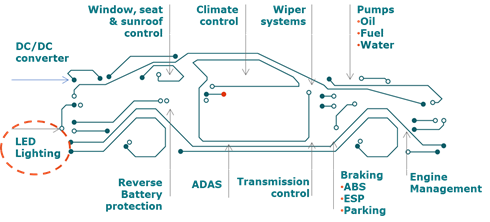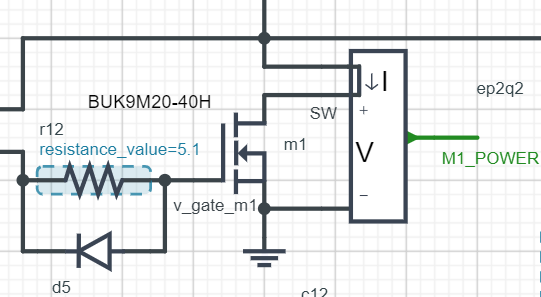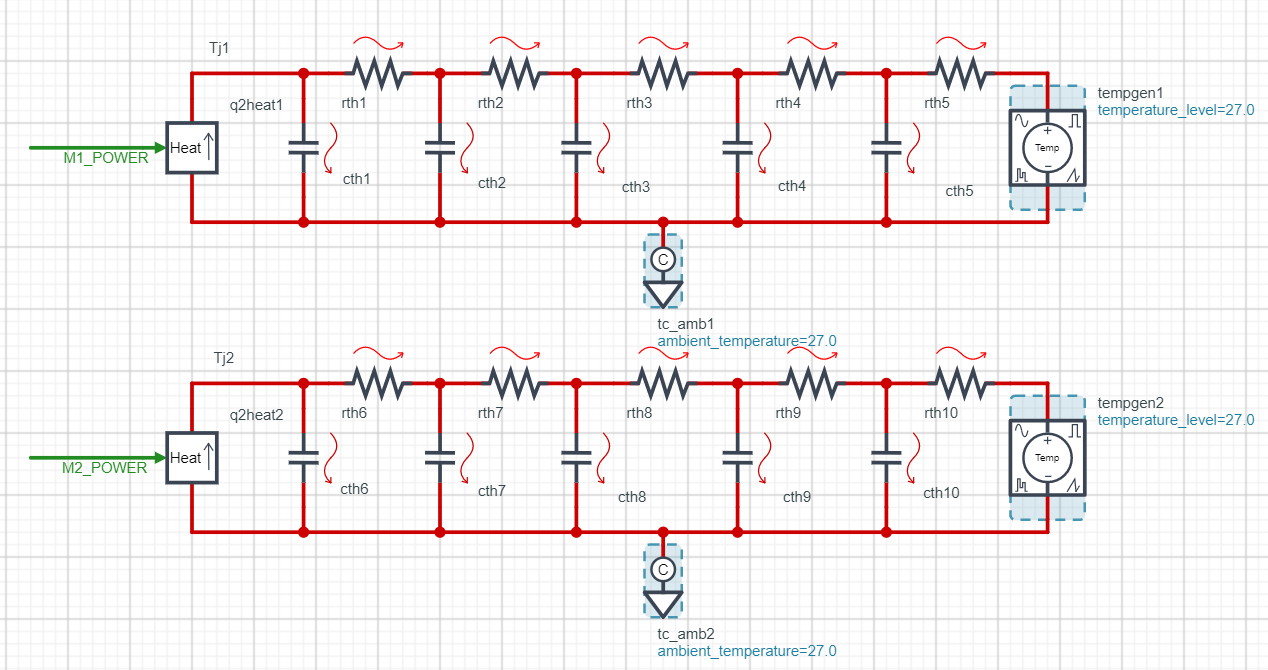# IAN50002 - LED side light SEPIC DC-to-DC converter

This interactive application note provides a general guideline of Single-Ended Primary Inductance Converter (SEPIC) DC-to-DC converter design using Nexperia Power MOSFET devices.

Authors: Mark Fang, Phil Ellis and Mike Zeng, Automotive Application Engineers, Shanghai & Manchester

In this interactive application note, a general guideline of Single-Ended Primary Inductance Converter (SEPIC) DC-to-DC converter design using Nexperia power MOSFET devices will be described. The traditional method is using theoretical calculation. This method has limitations as thermal calculation and power device junction temperature estimation are difficult with complex current waveforms. For optimization of the system, several calculation iterations are required. Full system design can be simulated directly through an embedded simulator available on this page.

This interactive application note contains embedded SystemVision® Cloud simulations to augment the text.

To open the embedded simulation, simply hover over the simulation image. Left click anywhere in the graphic area once the central play button changes in colour from black to yellow. This opens the schematic in the SystemVision® Cloud environment. Some of the components have a blue highlight, right click and select “properties” to choose  the most appropriate value for the simulation, these might be limited to a certain range or selected from a dropdown list. To run the simulation, press the large green play button at the top of the schematic. Key probe points are included, you can add further probes using the probe tool and change the simulation settings using the gear wheel button grouped with the large green play  button. See accompanying application note: AN50002

### 1. Introduction

In modern automotive applications, electrical systems are widely used in vehicles. They usually powered by lead-acid or Li-ion batteries whose output voltage varies with the State of Charge (SOC), Temperature, Load Dump and other loading operations. This requires DC-to-DC converters to accommodate a wide input voltage and generate a stable regulated output voltage for electrical loads. A Single-Ended Primary Inductance Converter (SEPIC) design can be a good fit for a battery powered system. It can generate output voltage higher or lower than the input voltage with less components comparing to other topologies. Additionally, its AC coupled capacitor inherently separates the output from the input. LED Lighting is one example where SEPIC converters can be used in an automotive application.Figure 1. Automotive Application -  LED Lighting.

In this interactive application note, a general guideline of SEPIC converter design using Nexperia Power MOSFET devices will be described. System design can be simulated directly through an embedded simulator.

### 2. SEPIC converter design simulation

A synchronous SEPIC converter design example is included in the simulation below. The typical waveforms of each voltage node and current through each device can be viewed by the Waveform Viewer in simulator below. For example, the VDS and VGS of the main control MOSFET is shown in the purple and brown waveforms. The blue waveform demonstrates output voltage ramping up gradually due to the soft-start function of the controller. More waveform viewer can be added from tool bar next to the ‘Run’ button. Additionally, the parameters of the components with blue dashed frames can be tuned by the user for desired system configuration or different operation points.

Simulation 1. Synchronous SEPIC converter design. Click arrow to open the simulation.

One of the features of SEPIC converters is that the currents flowing through the two inductances are proportional to each other. Hence, a coupled inductor can be used in the design for reducing PCB footprint size and system cost.

Example design parameters:

• Input voltage: 9 -16 V
• Output Voltage: 20 V
• Maximum output power: 20 W
• Switching frequency: 380 kHz

Key features:

• Reverse battery protection (RBP)
• Synchronous topology improves converter efficiency
• Configurable light load operation modes

### System design

SEPIC topology is a combined topology consisting of two building blocks as shown in Fig. 2 below.

At the input side, there is a common Boost configuration formed by an inductor and a power switch. This eases the RMS current seen by input capacitor.

At the output side, a Buck block is formed by a synchronous MOSFET or diode and an inductor. As these two inductors are being charged and discharged during the whole operation period, a coupled inductor can be used to reduce size and cost.

With this configuration, a SEPIC converter is able to accommodate a wide input voltage range and provide a regulated output.Figure 2. SEPIC converter building blocks

To ensure proper energy transfer and operation of a SEPIC converter, a coupling capacitor with low ESR is required. The RMS current rating of this capacitor is proportional to output power, since all output current is passing through coupling capacitor. And maximum input voltage plus some ripple will determine the voltage rating. This capacitor is also the inter-connection between two building blocks.

### Basic operation

The basic operation of a SEPIC converter is shown in Fig. 3 (a) below.

During the period that switch Q1 = ON the coupled inductor (two windings) and coupling capacitor are being charged and energy is stored inside them. Current flow is indicated in Fig. 3 (b). In this period, all output power is provided by output capacitor to maintain regulated output voltage rating.

When Q1 = OFF, and synchronous MOSFET Q2 = ON, Fig. 3 (c), energy is released from inductors and delivered to the load.a) simplified schematicb) Q1 = ON; Q2 = OFFc) Q1 = OFF; Q2 = ON

Figure 3. SEPIC converter phases

### 3. Power MOSFET selection

Power MOSFET selection plays a critical part for the whole design as it determines the whole system’s thermal design requirements, cost and efficiency. Theoretical analysis can be performed with mathematical equations. In SEPIC topology, the voltage stress on main and synchronous MOSFET is the sum of maximum input voltage and regulated output voltage:During the main switch on state, both inductor currents will pass through the main control MOSFET. The peak and RMS current can be calculated:During the main switch off state, both inductor current will through the synchronous MOSFET, consequently the peak and RMS current are expressed as:With current and voltage rating known, we can calculate the switching and conduction losses for the main MOSFET:Where ΔVis gate voltage sweeping range, and Ciss is the MOSFET input parasitic capacitance, Ig is the gate drive sinking and sourcing current.

And for synchronous MOSFET the switching and conduction losses can be obtained by:During converter design, theoretical analysis utilizes complicated mathematical equations. Some of these can be only approximations. For instance, the entire switching process of a MOSFET is non-linear. There is always some discrepancy between theorical calculation results and the measured ones. In addition, in thermal aspect only steady state junction temperature can be estimated through mathematical equations. It is very difficult to estimate instantaneous junction temperature through theoretical equations since the current profile could be very complex. Several iterations of calculation are usually needed to choose the right MOSFET that meets the system efficiency, cost and size requirement. An easier and more advanced approach is to use an embedded simulation tool with Nexperia MOSFET model.

Simulation 2. SEPIC Converter with thermal model. Click arrow to simulate.

In simulation domain, power dissipation in a MOSFET can be easily measured with the Power Dissipation Monitor shown in Fig. 4. The monitor is placed in series with the MOSFET, therefore continuously monitoring the current through the MOSFET. It also measures the voltage across MOSFET. Consequently, power dissipation can be calculated inside the model and output as a quantity value. This instantaneous power rating can be directly shown in the waveform viewer ‘m1_power’.Figure 4. Power Dissipation Monitor

The output of the Power Dissipation Monitor will work with a Cauer type thermal RC network model shown in Fig. 5 to give a junction temperature estimation. The ‘tempgen1’ and ‘tempgen2’ set the differential initial mounting base temperature from system ambient temperature. The ambient temperature is set by ‘tc_amb1’ and ‘tc_amb2’. All temperature setting should be within -55~175℃.Figure 5. Cauer type Thermal RC Network

### 4. Other design consideration

The following consideration is based on SEPIC converter operating in Continuous Conduction Mode (CCM) .

4.1 Duty Cycle

In CCM operation, the duty cycle of a SEPIC converter can be obtained as:Where VD is the voltage across the diode or the synchronous MOSFET.

4.2 Inductor selection

There are several factors affecting the choice of inductance value like RMS current, peak-to-peak current ripple, current to ripple ratio, maximum input current (at minimum Vin), and switching frequency. One of the important factors among them is the current ripple ratio (r) which is defined as the ratio between inductor ripple current and average current. As a rule of thumb, the r value of 0.4 would be a good starting point . A higher value will bring high stress to the input capacitor (see input capacitor selection in later section.) A lower value will require higher inductor energy handling capability, which means thicker wire and larger physical size. Therefore, an inductor value can be calculated by the following equations:In practice, inductor RMS and peak current should also be considered to ensure proper operation and design efficiency. They can be obtained by:4.3 Bootstrap circuit design

In order to fully turn-on synchronous high side MOSFET, a floating power supply rail is required to provide at least 5 V above the MOSFET source voltage level. A bootstrap circuit formed by a capacitor, a diode and a series resistor (limiting the capacitor charging inrush current) is often used in practice. The capacitance value should be selected according to MOSFET gate charge requirement. In other words, this capacitor should provide full charge to the MOSFET gate.

4.4 AC coupling capacitor

One of the distinguishing advantages of a SEPIC converter is that input and output terminal is separated by the AC coupling capacitor. However, the RMS rating of this capacitor is proportional to the output power. Hence, this topology is mainly used in low and medium power application. During main control MOSFET turn-on period, IL2 pass through coupling capacitor . In turned-off period, IL1 goes through coupling capacitor . Therefore, the RMS current and capacitance of AC coupling capacitor can be obtained by following equations.Where IL1 and IL2 are average current of the inductor.

In addition, when the primary control switch is on, this coupling capacitor will be charged to input voltage and some added ESR ripple. Therefore, the voltage rating should be higher than the maximum input voltage.

4.5 Input and output capacitor

The SEPIC is a combined topology. The input side has an inductor like a boost converter. Due to the existence of this inductor, the input current is triangular and continuous. Therefore, the RMS current of the input capacitor can be obtained by following equation. The voltage rating should be higher than the maximum input voltage.As the output capacitor is required to provide charge to the load during control MOSFET on state. Large ripple current would be seen by the output capacitor. Hence, RMS current should be calculated:In practice, any parasitic parameters like ESR or ESL of a bulk capacitor will contribute to output voltage ripple. Thus, the output capacitor should be selected according to converter output ripple requirements. The relationship between output voltage ripple and ESR as well as capacitance is described by the following equations:4.6 Output voltage

The output voltage can be set easily through the ratio of a potential divider. The internal feedback reference voltage is 1.2 V.4.7 Soft start-up

A soft start-up operation is realized by using a capacitor connected to SS pin of the controller. An internal current charger of 10 μA will continuously charge this capacitor until the voltage reaches the preset feedback threshold value of 1.2 V.### 5. Conclusion

In this interactive application note a general design approach of a SEPIC DC-to-DC converter is presented. The traditional method is using theoretical calculation. This method has limitations as thermal calculation and power device junction temperature estimation are difficult with complex current waveforms. For optimization of the system, several calculation iterations are required. A more advanced design approach is using Nexperia pre-define simulation model in SystemVision.

SystemVision® embedded Cloud simulations were used in this interactive application note.

### 6. Reference

 Switching Power Supplies A-Z, Second Edition – Sanjaya Maniktala

 Page last updated 05 May 2021.